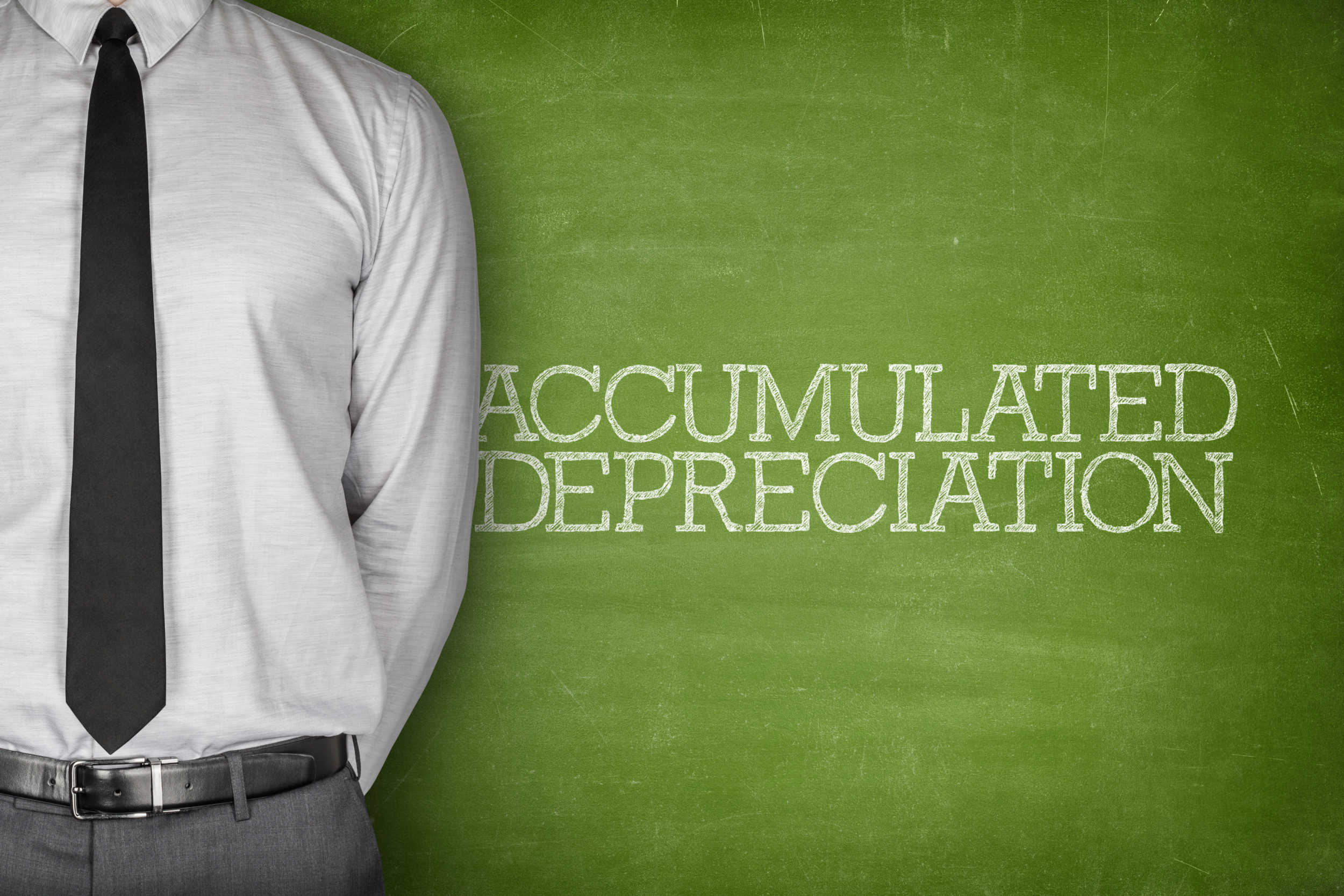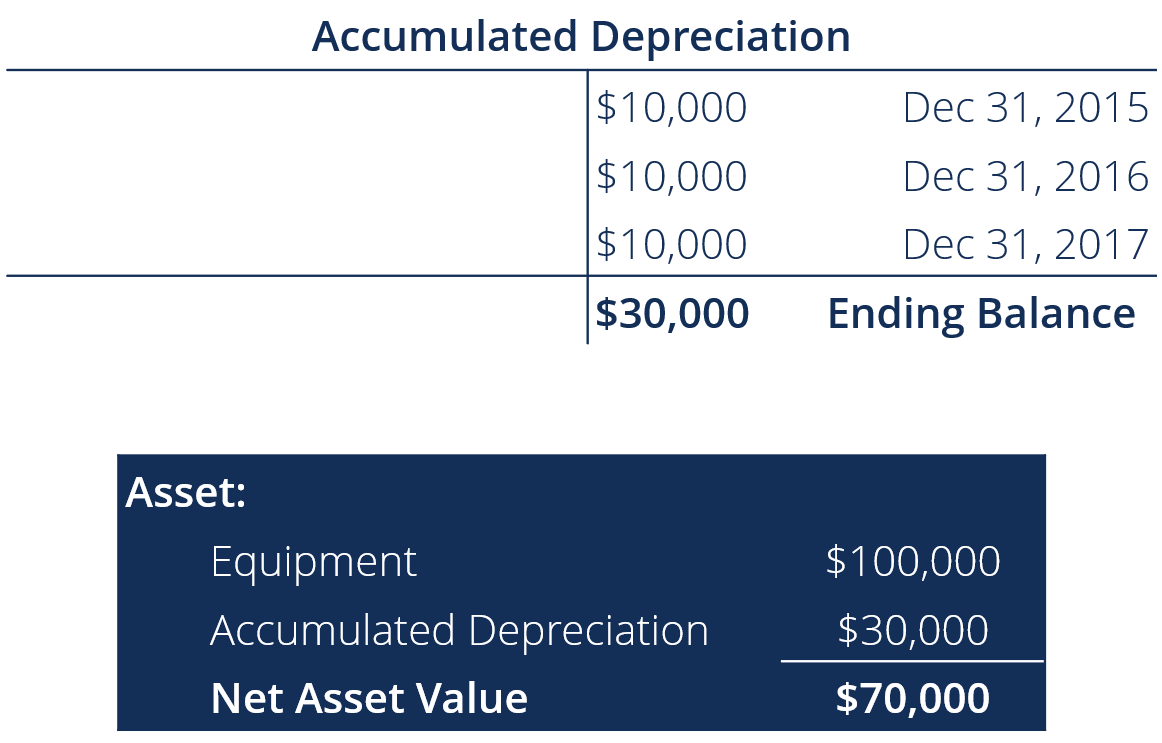# Accumulated Depreciation

Total depreciation over an asset's life

## What is Accumulated Depreciation?

Accumulated depreciation is the total amount of depreciation expense allocated to a specific asset since the asset was put into use. It is a contra-asset account – a negative asset account that offsets the balance in the asset account it is normally associated with.

Unlike a normal asset account, a credit to a contra-asset account increases its value while a debit decreases its value. Whenever depreciation expense is recorded for an organization, the same amount is also credited to the accumulated depreciation account, allowing the company to show both the cost of the asset and total depreciation of the asset. This also shows the asset’s net book value on the balance sheet.

Financial analysts will create a depreciation schedule when performing financial modeling to track the total depreciation over an asset’s life.### Example

XYZ Company purchased equipment on January 1, 2015 for \$100,000. The equipment has a residual value of \$20,000 and has an expected useful life of 8 years. On December 31, 2017, what is the balance of the accumulated depreciation account?

(\$100,000 – \$20,000) / 8 = \$10,000 in depreciation expense per year### Debiting Accumulated Depreciation

In most scenarios, we credit the accumulated depreciation account because, as time passes, the company records the depreciation expense that is accumulated in the contra-asset account. However, there are situations when the accumulated depreciation account is debited or decreased. For example, let’s say an asset has been used for 5 years and has an accumulated depreciation of \$100,000 in total. After the 5-year period, if the company were to sell the asset, the account would need to be zeroed out because the asset is not relevant to the company anymore. Therefore, there would be a credit to the asset account, a debit to the accumulated depreciation account, and a gain or loss depending on the fair value of the asset and the amount received.

### Accumulated Amortization/Depletion

Accumulated amortization and accumulated depletion work in the same way as accumulated depreciation; they are all contra-asset accounts. The naming convention is just different depending on the nature of the asset. For tangible assets such as property or plant and equipment, it is referred to as depreciation. For intangible assets such as patents, licenses, or trademarks, it is referred to as amortization, and for natural resources-related assets such as mines or oil platforms, depletion is the official terminology. When amortization or depletion expense is recorded for the year, the corresponding accumulated contra-asset accounts are credited in order to account for the expense.

We hope you enjoyed reading our explanation of accumulated depreciation. CFI offers a wealth of free resources on financial analysis and accounting, including the following:

• Depreciation Expense
• Depreciation Schedule
• Projecting Income Statement Line Items
• Income Statement Template